## Kaggle’s “Human or Robot?” competition feedbackA month ago, I started participating in my first Kaggle competition. I wanted to start participating in Kaggle competitions for a while and Facebook launched a recruiting competition: Human or Robot?, and I decided to join the party.

I was finally ranked 32nd on the final ranking (private learderboard), and I could actually have been ranked 17th if I had chosen another of my predictions as final submission… (That additionnal URL feature wasn’t that useless afterall…)

This blog post is a (slightly modified) export of my IPython notebooks written for this competition. Well… Let’s get started!

TL;DR: I used a bunch of basic statistical features extracted from aggregation of bids of each bidder and trained a Random Forest tuned by 10-fold-CV. Plain and simple.

# Import needed libraries for dataset creation

Nothing fancy here, we just use some classical Python Data Science libraries: numpy, scikit-learn and pandas, plus pickle to save the result.

import pickle
import numpy as np
import sklearn.preprocessing
from sklearn_pandas import DataFrameMapper
import pandas as pd


First step is to read the CSV files and load them as Pandas frames. I used Pandas here because of the “heavy” work needed to create the features, being very easy to do with Pandas and that would have been much more painful to do with numpy only for example.

# Read the bids, replace NaN values with a dash because NaN are string categories we don't know
bids.fillna('-', inplace=True)

# Load train and test bidders lists (nothing really interesting in that list)

# Join those 2 datasets together (-1 outcome meaning unknown i.e. test)
test['outcome'] = -1.0
bidders = pd.concat((train, test))


# Dataset investigation

Prior to the feature creation, some inspection on the data has been done, of course, but I didn’t kept any trace of this quick’n’dirty work anywhere and won’t be able to show it to you.

The idea is to get to know what’s in the dataset. The first step is just to show look at the raw data, and to then look at some stats about the data. For example, the first thing you might wonder is what the heck can I do with those payment_account and address hashes that Kaggle gave me for each bidder. Is there any of these things that appear more than once? Nope, they are as unique as the bidder_id so you can throw this away. At this moment you know that all your features must come from the bids themselves.

Then you do some stats and plots of what’s in the bids, you look what could be interesting to compute features…

# Features creation

… and then there is a moment when you decide that it’s time to get this party started and start to do something with these bids.

The things I hesitated about was to decide if I should predict if a bidder is a bot based on aggregates of info about his bids, or if I should predict if a bid has been made by a bot for each bid and then aggregate the predictions at bid level to create a prediction at bidder level.

I preferred to work at the bidder level because I had the feeling that each bid don’t have enough info by itself to allow proper prediction, and that the aggregation of a set of bids would allow me to create more high level features about the general behavior of a bidder and therefore get more useful info.

I didn’t have the time to actually try the bid-level prediction approach. I don’t know how it would have turned out.

So the first thing you do is to look at the info you have on each bid and look at what feature you can compute with this. We have this:

• auction (category) – Unique identifier of an auction
• merchandise (category) – The category of the auction site campaign, which means the bidder might come to this site by way of searching for “home goods” but ended up bidding for “sporting goods” – and that leads to this field being “home goods”. This categorical field could be a search term, or online advertisement.
• device (category) – Phone model of a visitor
• time (real) – Time that the bid is made (transformed to protect privacy).
• country (category) – The country that the IP belongs to
• ip (category) – IP address of a bidder (obfuscated to protect privacy).
• url (category) – url where the bidder was referred from (obfuscated to protect privacy).

We only have one real feature and lots of categories.

My first approach was what I’ve seen called “kitchen sink approach”, I basically decided to compute whatever statistical computation crossed my mind (as long as I wasn’t too lazy to implement it so it had better be simple or genius). I decided to apply the same analysis on all categories. And the same idea goes for the real variable, with different stats of course.

## Category variable feature extraction

The idea is to group the bids of a bidder and compute stats about the group (which is therefore a series of value for each variable). Then for a list of string, you get stats about these strings and their frequencies:

• number of unique categories that appear
• highest frequency that appearance
• lowest frequency of appearance
• category that appear the most
• standard deviation of the frequencies

and… that’s it!

def computeStatsCat(series, normalizeCount = 1.0):

n = float(series.shape)
counts = series.value_counts()

nbUnique = counts.count() / normalizeCount
hiFreq = counts / n
loFreq = counts[-1] / n
argmax = counts.index
stdFreq = np.std(counts / n)

return (nbUnique, loFreq, hiFreq, stdFreq, argmax)


## Real variable feature extraction

For time, I decided to go a little bit deeper and group the series of timestamp of bids by auction and have two stages of stats, stats at auction level that are then aggregated and global stats for the whole set of timestamps of the bidder.

You see below a few functions that allowed me to compute those features. Basically, for each auction I compute stats that are: min, max, range of timestamps, and then I compute a bunch of things about interval between two bids: mean interval, standard deviation, percentiles. I then aggregate those results for all the auction a bidder had always in the same ideas of simple stats.

# Compute stats of numerical series without caring about interval between values
def computeStatsNumNoIntervals(series):

min = series.min()
max = series.max()
mean = np.mean(series)
std = np.std(series)
perc20 = np.percentile(series, 20)
perc50 = np.percentile(series, 50)
perc80 = np.percentile(series, 80)

return (min, max, mean, std, perc20, perc50, perc80)

# Compute stats of a numerical series, taking intervals between values into account
def computeStatsNum(series, copy = True):

if copy:
series = series.copy()

series.sort()
intervals = series[1:].as_matrix() - series[:-1].as_matrix()
if len(intervals) < 1:
intervals = np.array()

nb = series.shape
min = series.min()
max = series.max()
range = max - min
intervalsMin = np.min(intervals)
intervalsMax = np.max(intervals)
intervalsMean = np.mean(intervals)
intervalsStd = np.std(intervals)
intervals25 = np.percentile(intervals, 25)
intervals50 = np.percentile(intervals, 50)
intervals75 = np.percentile(intervals, 75)

return (nb, min, max, range,
intervalsMin, intervalsMax, intervalsMean, intervalsStd,
intervals25, intervals50, intervals75)

# Compute stats about a numerical column of table, with stats on sub-groups of this column (auctions in our case).
def computeStatsNumWithGroupBy(table, column, groupby):

# get series and groups
series = table[column]
groups = table.groupby(groupby)

# global stats
(nb, min, max, range,
intervalsMin, intervalsMax, intervalsMean, intervalsStd,
intervals25, intervals50, intervals75) = computeStatsNum(series)

# stats by group
X = []
for _, group in groups:
(grpNb, _, _, grpRange, grpIntervalsMin, grpIntervalsMax, grpIntervalsMean, grpIntervalsStd, _, _, _) = computeStatsNum(group[column])
X.append([grpNb, grpRange, grpIntervalsMin, grpIntervalsMax, grpIntervalsMean, grpIntervalsStd])
X = np.array(X)

grpNbMean = np.mean(X[:,0])
grpNbStd = np.std(X[:,0])
grpRangeMean = np.mean(X[:,1])
grpRangeStd = np.std(X[:,1])
grpIntervalsMinMin = np.min(X[:,2])
grpIntervalsMinMean = np.mean(X[:,2])
grpIntervalsMaxMax = np.max(X[:,3])
grpIntervalsMaxMean = np.mean(X[:,3])
grpIntervalsMean = np.mean(X[:,4])
grpIntervalsMeanStd = np.std(X[:,4])
grpIntervalsStd = np.mean(X[:,5])

return (nb, min, max, range,
intervalsMin, intervalsMax, intervalsMean, intervalsStd,
intervals25, intervals50, intervals75,
grpNbMean, grpNbStd, grpRangeMean, grpRangeStd,
grpIntervalsMinMin, grpIntervalsMinMean, grpIntervalsMaxMax, grpIntervalsMaxMean,
grpIntervalsMean, grpIntervalsMeanStd, grpIntervalsStd)


## Feature tried that did not really worked

### From categories to real values

In a desperate attempt to increase my score, I though about replacing categories in the bids dataset by real values by computing general stats about each category of each variable, and replacing this category by stats about this category, in my case the probability of this category to belong to appear in a bot’s bid.

I am aware that this is getting close to the danger of Data Leakage because you are explicitly introducing information about the target in the features. However, I feel like because the real value you use to represent the category is computed on the whole dataset, it might in some cases be ok because it is a very aggregated info, provided you have a lot of data in each category.

In this case, I think that if was definitely a data leakage because I got a 0.97 AUC on my CV but a 0.86 score on public leaderboard (a big drop from my results without those features). But it was worth trying!

def computeOutcomeProbaByCat(data, cats):
stats = {}
for cat in cats:
stats[cat] = pd.DataFrame(data.groupby(cat).aggregate(np.mean).outcome)
stats[cat].rename(columns={'outcome': cat+'Num'}, inplace=True)
return stats

#bidsWithOutcome = pd.merge(bids, bidders[['outcome']], how='left', left_on='bidder_id', right_index=True)
#stats = computeOutcomeProbaByCat(bidsWithOutcome[bidsWithOutcome.outcome >= 0], [u'auction', u'merchandise', u'device', u'country', u'url'])

# Add real columns to bids dataframe
#for cat in stats:
#    bids = pd.merge(bids, stats[cat], how='left', left_on=cat, right_index=True)


### Make a special case for merchandise category

I also wanted to make a special case for merchandise category, and have a couple of features per category indicating in a way how the bidder participated in the auctions of this merchandise: the number of bids in the category and the percentage of his bids made in this category.

This did not changed my score in any way, probably due to the fact that very few bidders actually participate in multiple merchandises if I remember well.

## Features I didn’t tried

There are a lot of features I could have tried if I had time and motivation. You will find a lot of different things in others feedback from this contest. There are a lot of great and nice features I do not have, however, it seems that what I got here already gives you pretty good results.

Lots of people like to look at the contribution of each feature in the final classifiers. I’m sure it might give some information and ideas about how to improve your features. I didn’t do it in this competition. And I’m anyway not a big fan of interpreting a Machine Learning model, something also “criticized” in the great kdnuggets blog article “The Myth of Model Interpretability”.

## Global computation of features

Well, finally we need to compute all those features from our dataset so, I know this block of code is kind of dirty, but since I wanted to be able to include of exclude features at ease, this was my solution. This is the moment when multi-cursors feature of Sublime Text takes stats being very useful.

# Init vars
Xids = []
X = []

# merchandises = bids.merchandise.value_counts()

# For each bidder
for bidder, group in bids.groupby('bidder_id'):

# Compute the stats
(nbUniqueIP, loFreqIP, hiFreqIP, stdFreqIP, IP) = computeStatsCat(group.ip)
(nbUniqueDevice, loFreqDevice, hiFreqDevice, stdFreqDevice, device) = computeStatsCat(group.device)
(nbUniqueMerch, loFreqMerch, hiFreqMerch, stdFreqMerch, merch) = computeStatsCat(group.merchandise)
(nbUniqueCountry, loFreqCountry, hiFreqCountry, stdFreqCountry, country) = computeStatsCat(group.country)
(nbUniqueUrl, loFreqUrl, hiFreqUrl, stdFreqUrl, url) = computeStatsCat(group.url)
(nbUniqueAuction, loFreqAuction, hiFreqAuction, stdFreqAuction, auction) = computeStatsCat(group.auction)
(auctionNb, auctionMin, auctionMax, auctionRange,
auctionIntervalsMin, auctionIntervalsMax, auctionIntervalsMean, auctionIntervalsStd,
auctionIntervals25, auctionIntervals50, auctionIntervals75,
auctionGrpNbMean, auctionGrpNbStd, auctionGrpRangeMean, auctionGrpRangeStd,
auctionGrpIntervalsMinMin, auctionGrpIntervalsMinMean, auctionGrpIntervalsMaxMax, auctionGrpIntervalsMaxMean,
auctionGrpIntervalsMean, auctionGrpIntervalsMeanStd, auctionGrpIntervalsStd) = computeStatsNumWithGroupBy(group, 'time', 'auction')

# Save the stats
# Also I don't really remember which category features I kept or not in my final submission :\$
# I think it was IP + device + merch + contry, but for computation time let's comment some of these
x = [nbUniqueIP, loFreqIP, hiFreqIP, stdFreqIP, #IP,
nbUniqueDevice, loFreqDevice, hiFreqDevice, stdFreqDevice, #device,
nbUniqueMerch, loFreqMerch, hiFreqMerch, stdFreqMerch, merch,
nbUniqueCountry, loFreqCountry, hiFreqCountry, stdFreqCountry, country,
nbUniqueUrl, loFreqUrl, hiFreqUrl, stdFreqUrl, #url,
nbUniqueAuction, loFreqAuction, hiFreqAuction, stdFreqAuction, #auction
auctionNb, auctionMin, auctionMax, auctionRange,
auctionIntervalsMin, auctionIntervalsMax, auctionIntervalsMean, auctionIntervalsStd,
auctionIntervals25, auctionIntervals50, auctionIntervals75,
auctionGrpNbMean, auctionGrpNbStd, auctionGrpRangeMean, auctionGrpRangeStd,
auctionGrpIntervalsMinMin, auctionGrpIntervalsMinMean, auctionGrpIntervalsMaxMax, auctionGrpIntervalsMaxMean,
auctionGrpIntervalsMean, auctionGrpIntervalsMeanStd, auctionGrpIntervalsStd]

## Old stats per merchandise
# for key in merchandisesCounts.index:
#     merchandisesTmp[key] = merchandisesCounts[key]
#     merchandisesTmp2[key] = float(merchandisesCounts[key]) / len(group)
# merchandisesTmp = merchandises * 0
# merchandisesTmp2 = (merchandises * 0).astype('float')
# merchandisesCounts = group.merchandise.value_counts()
# x += merchandisesTmp.tolist();
# x += merchandisesTmp2.tolist();

# Old stats replacing using real value substitution of categories
# catCols = []
# for cat in stats:
#     (catMin, catMax, catMean, catStd, catPerc20, catPerc50, catPerc80) = computeStatsNumNoIntervals(group[cat+'Num'])
#     x += [catMin, catMax, catMean, catStd, catPerc20, catPerc50, catPerc80]

# Save the stats in the result arrays
Xids.append(bidder)
X.append(x)

# Features labels
Xcols = ['nbUniqueIP', 'loFreqIP', 'hiFreqIP', 'stdFreqIP', #'IP',
'nbUniqueDevice', 'loFreqDevice', 'hiFreqDevice', 'stdFreqDevice', #'device',
'nbUniqueMerch', 'loFreqMerch', 'hiFreqMerch', 'stdFreqMerch', 'merch',
'nbUniqueCountry', 'loFreqCountry', 'hiFreqCountry', 'stdFreqCountry', 'country',
'nbUniqueUrl', 'loFreqUrl', 'hiFreqUrl', 'stdFreqUrl', #'url',
'nbUniqueAuction', 'loFreqAuction', 'hiFreqAuction', 'stdFreqAuction','auctionNb', 'auctionMin', 'auctionMax', 'auctionRange',
'auctionIntervalsMin', 'auctionIntervalsMax', 'auctionIntervalsMean', 'auctionIntervalsStd',
'auctionIntervals25', 'auctionIntervals50', 'auctionIntervals75',
'auctionGrpNbMean', 'auctionGrpNbStd', 'auctionGrpRangeMean', 'auctionGrpRangeStd',
'auctionGrpIntervalsMinMin', 'auctionGrpIntervalsMinMean', 'auctionGrpIntervalsMaxMax', 'auctionGrpIntervalsMaxMean',
'auctionGrpIntervalsMean', 'auctionGrpIntervalsMeanStd', 'auctionGrpIntervalsStd']

# Old features labels when replacing using real value substitution of categories
# for cat in stats:
#     Xcols += [cat + 'NumMin', cat + 'NumMax', cat + 'NumMean', cat + 'NumStd', cat + 'NumPerc20', cat + 'NumPerc50', cat + 'NumPerc80']
# Xcols += map(lambda x: "merch" + x + "Abs", merchandisesTmp.keys().tolist())
# Xcols += map(lambda x: "merch" + x + "Prop", merchandisesTmp.keys().tolist())

# Create a pandas dataset, remove NaN from dataset and show it
dataset = pd.DataFrame(X,index=Xids, columns=Xcols)
dataset.fillna(0.0, inplace=True)
dataset

nbUniqueIP loFreqIP hiFreqIP stdFreqIP nbUniqueDevice loFreqDevice hiFreqDevice stdFreqDevice nbUniqueMerch loFreqMerch auctionGrpNbStd auctionGrpRangeMean auctionGrpRangeStd auctionGrpIntervalsMinMin auctionGrpIntervalsMinMean auctionGrpIntervalsMaxMax auctionGrpIntervalsMaxMean auctionGrpIntervalsMean auctionGrpIntervalsMeanStd auctionGrpIntervalsStd
001068c415025a009fee375a12cff4fcnht8y 1 1.000000 1.000000 0.000000e+00 1 1.000000 1.000000 0.000000e+00 1 1 0.000000 0.000000e+00 0.000000e+00 0 0.000000e+00 0.000000e+00 0.000000e+00 0.000000e+00 0.000000e+00 0.000000e+00
002d229ffb247009810828f648afc2ef593rb 1 1.000000 1.000000 0.000000e+00 2 0.500000 0.500000 0.000000e+00 1 1 0.000000 1.052632e+08 0.000000e+00 105263158 1.052632e+08 1.052632e+08 1.052632e+08 1.052632e+08 0.000000e+00 0.000000e+00
0030a2dd87ad2733e0873062e4f83954mkj86 1 1.000000 1.000000 0.000000e+00 1 1.000000 1.000000 0.000000e+00 1 1 0.000000 0.000000e+00 0.000000e+00 0 0.000000e+00 0.000000e+00 0.000000e+00 0.000000e+00 0.000000e+00 0.000000e+00
003180b29c6a5f8f1d84a6b7b6f7be57tjj1o 3 0.333333 0.333333 0.000000e+00 3 0.333333 0.333333 0.000000e+00 1 1 0.000000 0.000000e+00 0.000000e+00 0 0.000000e+00 0.000000e+00 0.000000e+00 0.000000e+00 0.000000e+00 0.000000e+00
00486a11dff552c4bd7696265724ff81yeo9v 10 0.050000 0.300000 7.071068e-02 8 0.050000 0.350000 1.060660e-01 1 1 0.634324 1.518721e+12 2.301461e+12 0 1.090126e+12 5.571737e+12 1.401996e+12 1.246061e+12 1.775155e+12 1.559352e+11
ffbc0fdfbf19a8a9116b68714138f2902cc13 18726 0.000040 0.006341 1.099251e-04 792 0.000040 0.096989 4.973862e-03 1 1 197.065280 5.318327e+12 4.887044e+12 0 4.775914e+11 1.243363e+13 2.360377e+12 9.546782e+11 1.483847e+12 6.113005e+11
ffc4e2dd2cc08249f299cab46ecbfacfobmr3 18 0.045455 0.090909 1.889726e-02 13 0.045455 0.181818 4.504930e-02 1 1 0.805536 9.881656e+12 2.341891e+13 0 5.617653e+12 7.443268e+13 9.329175e+12 7.038926e+12 1.881787e+13 1.635072e+12
ffd29eb307a4c54610dd2d3d212bf3bagmmpl 1 1.000000 1.000000 0.000000e+00 1 1.000000 1.000000 0.000000e+00 1 1 0.000000 0.000000e+00 0.000000e+00 0 0.000000e+00 0.000000e+00 0.000000e+00 0.000000e+00 0.000000e+00 0.000000e+00
ffd62646d600b759a985d45918bd6f0431vmz 37 0.001506 0.055723 1.672626e-02 96 0.001506 0.301205 3.164567e-02 1 1 17.389500 4.444518e+12 4.925281e+12 0 1.002909e+11 9.788421e+12 1.723225e+12 5.569457e+11 7.713609e+11 5.499901e+11
fff2c070d8200e0a09150bd81452ce29ngcnv 1 1.000000 1.000000 0.000000e+00 1 1.000000 1.000000 0.000000e+00 1 1 0.000000 0.000000e+00 0.000000e+00 0 0.000000e+00 0.000000e+00 0.000000e+00 0.000000e+00 0.000000e+00 0.000000e+00

6614 rows × 48 columns

## Saving the final dataset

Now that we have a nice dataset, we need to make it a Machine Learnable one. Because we still have categories, we have variables that have a lot of different spans, etc. To do this, I used the DataFrameMapper class of sklearn-pandas package that allows you to easily transform a DataFrame into a numpy matrix of numbers.

# First lets join the dataset with outcome because it might be a useful info in the future ;)
datasetFull = dataset.join(bidders[['outcome']])
types = datasetFull.dtypes

# Create a mapper that "standard scale" numbers and binarize categories
mapperArg = []
for col, colType in types.iteritems():
if col == 'outcome':
continue
if colType.name == 'float64' or colType.name =='int64':
mapperArg.append((col, sklearn.preprocessing.StandardScaler()))
else:
mapperArg.append((col, sklearn.preprocessing.LabelBinarizer()))
mapper = DataFrameMapper(mapperArg)

# Apply the mapper to create the cdataset
Xids = datasetFull.index.tolist()
X = mapper.fit_transform(datasetFull)
y = datasetFull[['outcome']].as_matrix()

# Last check!
print bidders['outcome'].value_counts()

# Save in pickle file
pickle.dump([Xids, X, y], open('Xy.pkl', 'wb'))

-1    4700
0    1910
1     103
dtype: int64


# Learning to predict

Now that we have our dataset, let’s learn to predict bots!

Note: It is important to note that the performance results you see in this section are below my final score because to make this code more quickly runable I removed some of the categorical features that was used to get my final score.

## Useful imports and functions

As always, let’s start by importing the packages we need. Afterall, that’s how Python works, isn’t it? You want something, there’s a package for it…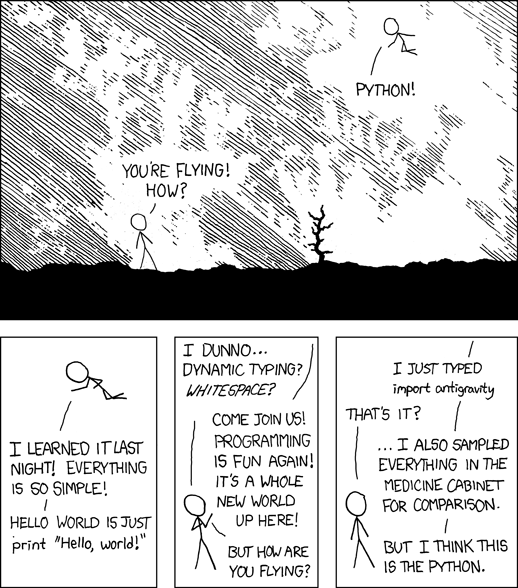# Data manipulation
import pickle
import pandas as pd
import numpy as np

# Machine Learning
import sklearn
import sklearn.ensemble
import sklearn.svm
from sklearn.cross_validation import train_test_split
from sklearn.grid_search import GridSearchCV
from sklearn.metrics import confusion_matrix
from sklearn.metrics import classification_report

# Plot
import matplotlib.pyplot as plt
import seaborn as sns
from pylab import rcParams
%matplotlib inline
%config InlineBackend.figure_format='retina'
rcParams['figure.figsize'] = 8, 5.5

# Utility functions

from sklearn.metrics import roc_curve, auc
from sklearn.preprocessing import label_binarize

# Plot a confusion matrix
def plotConfMap(confMat, classes=[], relative=False):

width = len(confMat)
height = len(confMat)

oldParams = rcParams['figure.figsize']
rcParams['figure.figsize'] = width, height

fig = plt.figure()
plt.clf()
plt.grid(False)
ax.set_aspect(1)

if not relative:
res = ax.imshow(confMat, cmap='coolwarm', interpolation='nearest')
else:
res = ax.imshow(confMat, cmap='coolwarm', interpolation='nearest', vmin=0, vmax=100)

for x in xrange(width):
for y in xrange(height):
ax.annotate(str(np.round(confMat[x][y], 1)), xy=(y, x),
horizontalalignment='center',
verticalalignment='center')

fig.colorbar(res)

if len(classes) > 0:
plt.xticks(range(width), classes)
plt.yticks(range(height), classes)

rcParams['figure.figsize'] = oldParams

return fig

# Plot CV scores of a 2D grid search
def plotGridResults2D(x, y, x_label, y_label, grid_scores):

scores = [s for s in grid_scores]
scores = np.array(scores).reshape(len(x), len(y))

plt.figure()
plt.imshow(scores, interpolation='nearest', cmap=plt.cm.RdYlGn)
plt.xlabel(y_label)
plt.ylabel(x_label)
plt.colorbar()
plt.xticks(np.arange(len(y)), y, rotation=45)
plt.yticks(np.arange(len(x)), x)
plt.title('Validation accuracy')

# Plot CV scores of a 1D "grid" search (a very narrow "grid")
def plotGridResults1D(x, x_label, grid_scores):

scores = np.array([s for s in grid_scores])

plt.figure()
plt.plot(scores)
plt.xlabel(x_label)
plt.ylabel('Score')
plt.xticks(np.arange(len(x)), x, rotation=45)
plt.title('Validation accuracy')


First, let’s load the dataset from our pickle file and split it into 3 sets:

• learn for the learning phase, split into:
• train for the training (will be used as validation with CV too)
• test to evaluate the results
• final for the final prediction, the one we will send to Kaggle
# Load
X_ids, X, y = pickle.load(open('Xy.pkl', 'rb'))
y = y.reshape(y.shape)
X_ids = np.array(X_ids)

# Split learn and final with outcome indices
i_final = (y == -1)
i_learn = (y > -1)

X_ids_final = X_ids[i_final]
X_ids_learn = X_ids[i_learn]
X_final = X[i_final, :]
X_learn = X[i_learn, :]
y_learn = y[i_learn]

# Split train and test
X_train, X_test, y_train, y_test = train_test_split(X_learn, y_learn, test_size=.25)


## RBF SVM

Having been taught Machine Learning in a large part by a researcher in SVM and kernel methods, my first Machine Learning try on a problem is often to use a SVM.

### Tuning the hyperparameters

To optimize the hyperparameters of my SVM, I’m going to do a double step grid search, first looking at the optimal value on a coarse grid, and then on a more fine grid around a promising area. You can actually do this a lot better, and especially you can accelerate the coarse grid a lot (that’s supposed to be the idea of it being coarse) for example by using a subset of the dataset. Here we might have dropped a lot of non-bots bidders I think. But anyway, that’s how it is…

One important thing is of course to think about finding the optimal value considering the AUC a scoring metrics, and not the classification rate. This obviously increase a lot your performance.

I chose to use 10 CV because my “bot” class contains really not a lot of values and I don’t want to drop to much of them in the test fold.

# Coarse grid
C_range = np.r_[np.logspace(-2, 20, 13)]
gamma_range = np.r_[np.logspace(-9, 5, 15)]
grid = GridSearchCV(sklearn.svm.SVC(C=1.0, kernel='rbf', class_weight='auto', verbose=False, max_iter=60000),
{'C' : C_range, 'gamma': gamma_range},
scoring='roc_auc', cv=10, n_jobs=8)
grid.fit(X_learn, y_learn)

plotGridResults2D(C_range, gamma_range, 'C', 'gamma', grid.grid_scores_)
plt.show()

# Display result
C_best = np.round(np.log10(grid.best_params_['C']))
gamma_best = np.round(np.log10(grid.best_params_['gamma']))
print 'best C coarse:', C_best
print 'best gamma coarse:', gamma_best

# Fine grid
C_range2 = np.r_[np.logspace(C_best - 1.5, C_best + 1.5, 15)]
gamma_range2 = np.r_[np.logspace(gamma_best - 1.5, gamma_best + 1.5, 15)]

gridFine = GridSearchCV(sklearn.svm.SVC(C=1.0, kernel='rbf', class_weight='auto', verbose=False, max_iter=60000),
{'C' : C_range2, 'gamma': gamma_range2},
scoring='roc_auc', cv=10, n_jobs=8)
gridFine.fit(X_learn, y_learn)

plotGridResults2D(C_range2, gamma_range2, 'C', 'gamma', gridFine.grid_scores_)

# Final result
bestClf = gridFine.best_estimator_
bestClf.probability = True
print bestClf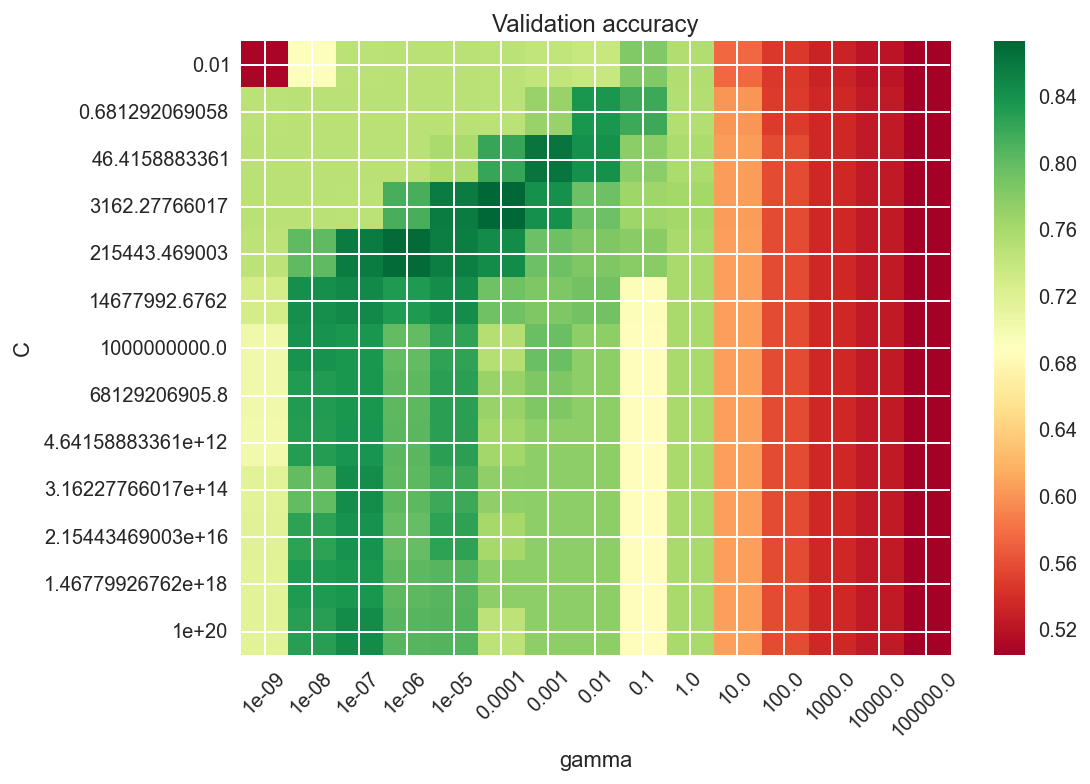best C coarse: 4.0
best gamma coarse: -4.0
SVC(C=517.94746792312128, cache_size=200, class_weight='auto', coef0=0.0,
degree=3, gamma=0.00043939705607607906, kernel='rbf', max_iter=60000,
probability=True, random_state=None, shrinking=True, tol=0.001,
verbose=False)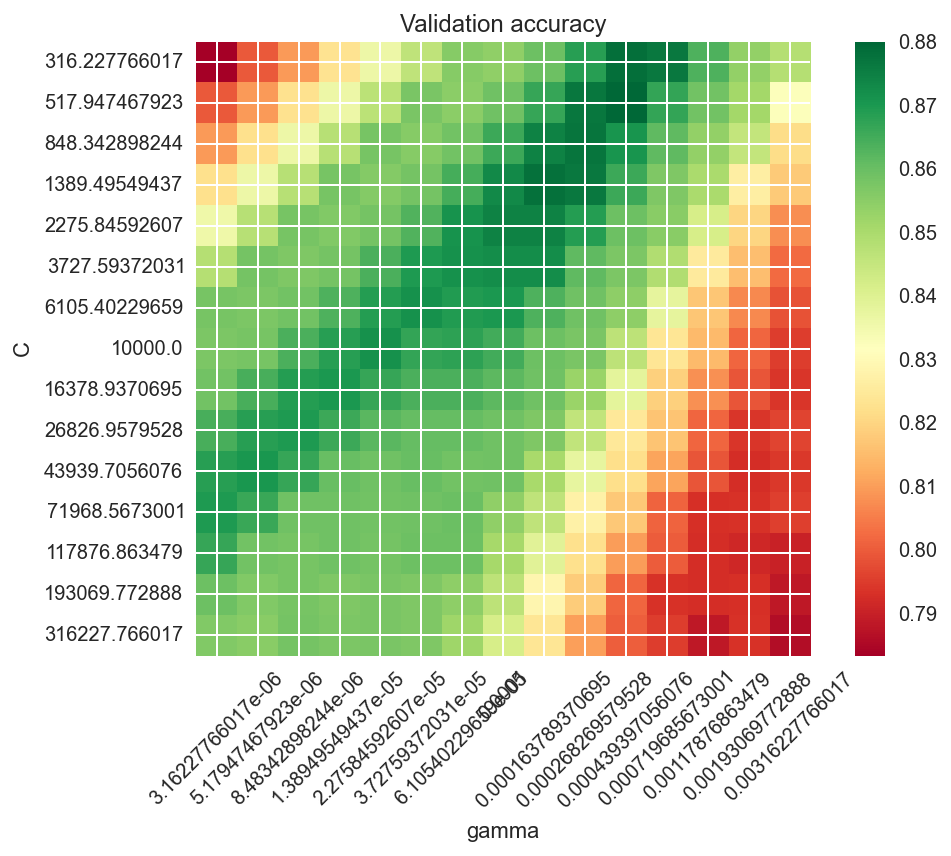### Testing the model

Now that we have our CV-optimized hyperparameters, let’s learn this classifier on the full train set and evaluate it on the test set.

# Fit it
bestClf.fit(X_train, y_train)
y_pred = bestClf.predict(X_test)

# Classif report and conf mat
print sklearn.metrics.classification_report(y_test, y_pred)
plotConfMap(sklearn.metrics.confusion_matrix(y_test, y_pred))
plt.show()

# Predict scores
y_score = bestClf.decision_function(X_test)

# Plot ROC
fpr, tpr, _ = roc_curve(y_test, y_score)
roc_auc = auc(fpr, tpr)

plt.figure()
plt.plot(fpr, tpr, label='ROC curve (area = %0.2f)' % roc_auc)
plt.plot([0, 1], [0, 1], 'k--')
plt.xlim([0.0, 1.0])
plt.ylim([0.0, 1.05])
plt.xlabel('False Positive Rate')
plt.ylabel('True Positive Rate')
plt.legend(loc="lower right")
plt.show()

             precision    recall  f1-score   support

0.0       0.97      0.82      0.89       464
1.0       0.20      0.62      0.30        32

avg / total       0.92      0.81      0.85       496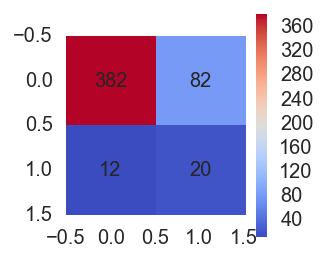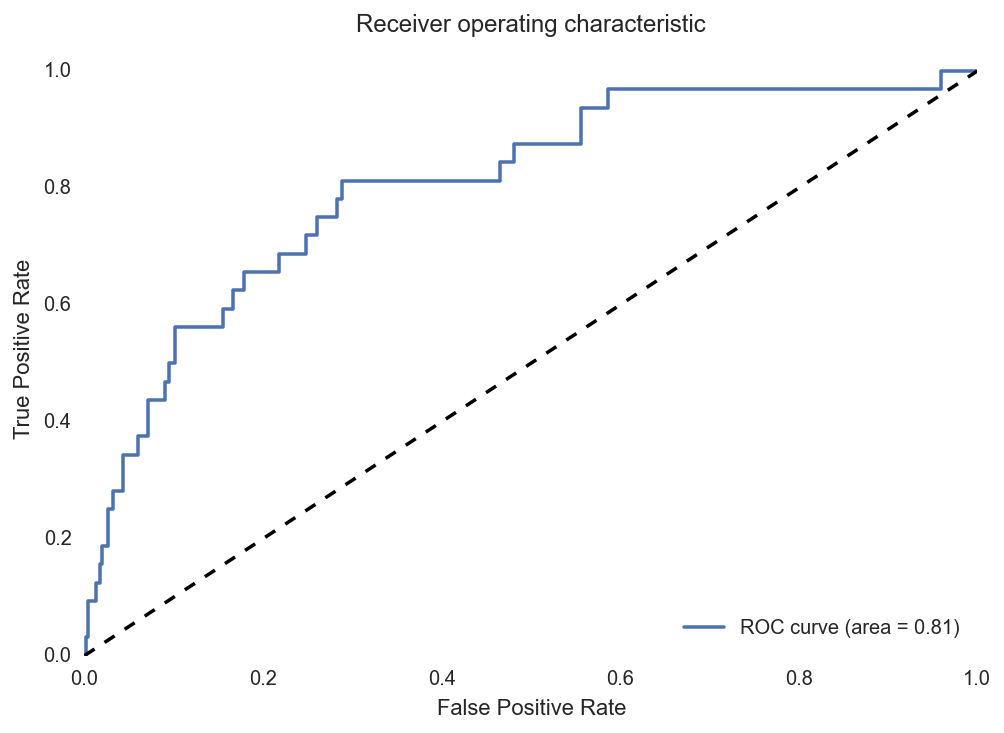### Final prediction

Ok, now let’s make our final prediction, learing on the full learning set and prediction on the final set.

# Fit on learn set and predict final set
bestClf.fit(X_learn, y_learn)
y_final = bestClf.predict_proba(X_final)[:,1]

# Dirty join with test.csv to order them like it was before...
# ... now that I look at this I am quite sure that Kaggle don't need them in the right
# order so all this is actually pretty stupid. Sorry :)
bidders_y_final = pd.DataFrame(np.c_[X_ids[i_final], y_final], columns=['bidder_id', 'prediction'])
bidders_y_final[['prediction']] = bidders_y_final[['prediction']].astype(float)
bidders_list_final = pd.merge(bidders_list[['bidder_id']], bidders_y_final, how='left').fillna(0.0)

# Write results to file
f = open('predictions_RBF_SVM.csv', 'wb')
f.write(bidders_list_final.to_csv(index=False))
f.close()


## Linear L1 SVM

Because of all the categories we kept from bids (highest frequent category use by a bidder, for a few variables), we have a lot of binary features, so it makes sense to use some L1 regularization. However, scikit-learn’s RBF SVM doesn’t have this feature, and I was too lazy to implement it myself so I tried the linear L1 SVM of scikit-learn. Also I wanted to try linear SVM as well.

The code is basically the same as for RBF SVM.

### Tuning the hyperparameters

# Coarse grid
C_range = np.r_[np.logspace(-2, 10, 12)]
grid = GridSearchCV(sklearn.svm.LinearSVC(C=1.0, penalty='l1', class_weight='auto', dual=False, verbose=False, max_iter=3000),
{'C' : C_range},
cv=10, n_jobs=8, scoring='roc_auc')
grid.fit(X_learn, y_learn)

plotGridResults1D(C_range, 'C', grid.grid_scores_)
plt.show()

# Results
C_best = np.round(np.log10(grid.best_params_['C']))
print 'best C coarse:', C_best

# Fine grid
C_range2 = np.r_[np.logspace(C_best - 1.5, C_best + 1.5, 15)]

gridFine = GridSearchCV(sklearn.svm.LinearSVC(C=1.0, class_weight='auto', dual=False, verbose=False, max_iter=3000),
{'C' : C_range2},
cv=10, n_jobs=8, scoring='roc_auc')
gridFine.fit(X_learn, y_learn)

plotGridResults1D(C_range2, 'C', gridFine.grid_scores_)

# Final results
bestClf = gridFine.best_estimator_
bestClf.probability = True
print bestClf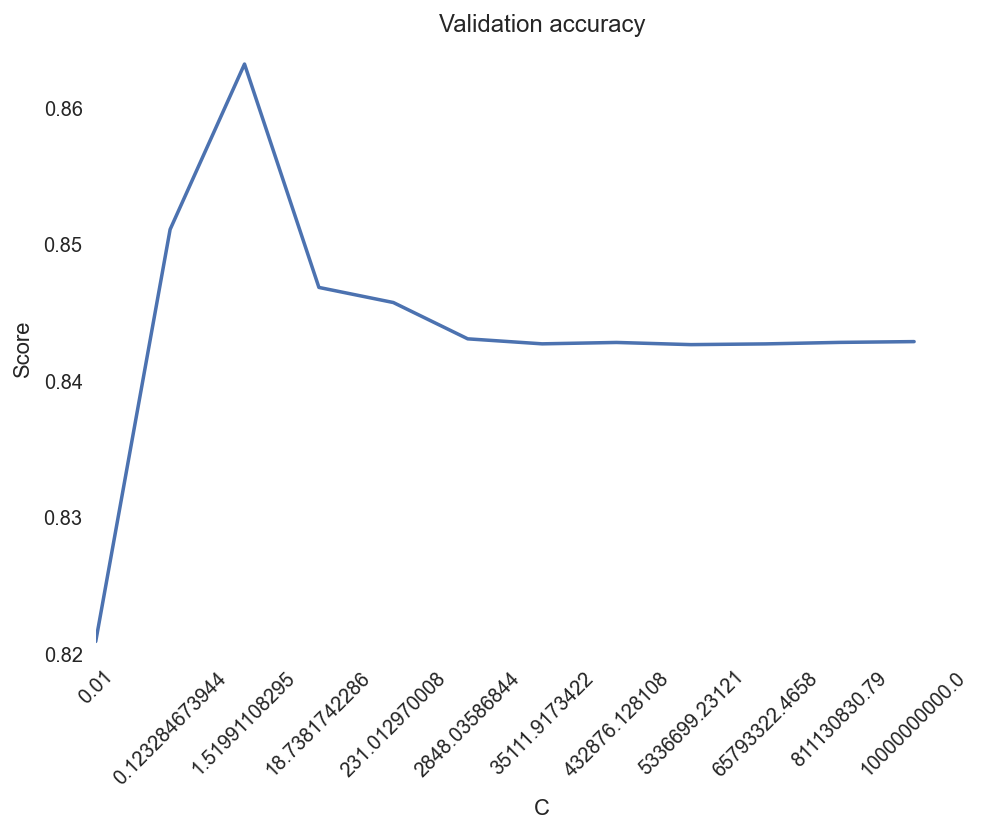best C coarse: 0.0
LinearSVC(C=0.13894954943731375, class_weight='auto', dual=False,
fit_intercept=True, intercept_scaling=1, loss='squared_hinge',
max_iter=3000, multi_class='ovr', penalty='l2', random_state=None,
tol=0.0001, verbose=False)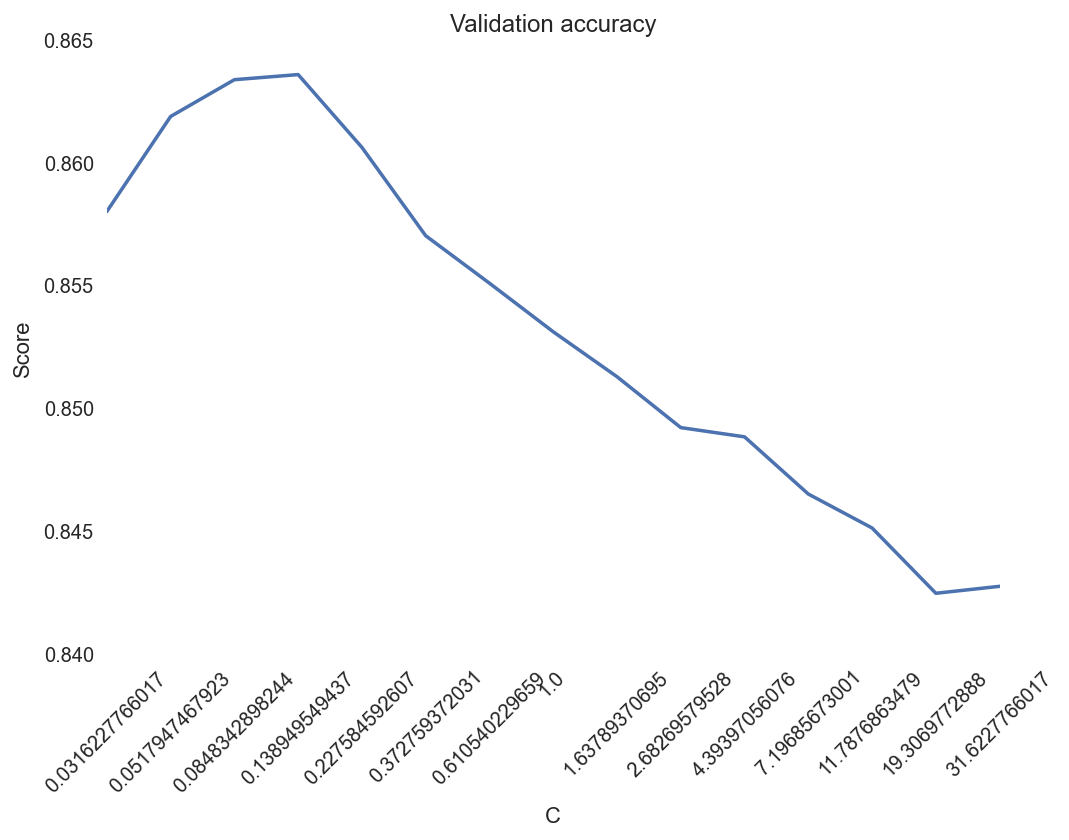### Testing the model

# Fit on train
bestClf.fit(X_train, y_train)
y_pred = bestClf.predict(X_test)

# Classification report
print sklearn.metrics.classification_report(y_test, y_pred)
plotConfMap(sklearn.metrics.confusion_matrix(y_test, y_pred))
plt.show()

# Predict
y_score = bestClf.decision_function(X_test)

# Plot ROC
fpr, tpr, _ = roc_curve(y_test, y_score)
roc_auc = auc(fpr, tpr)

plt.figure()
plt.plot(fpr, tpr, label='ROC curve (area = %0.2f)' % roc_auc)
plt.plot([0, 1], [0, 1], 'k--')
plt.xlim([0.0, 1.0])
plt.ylim([0.0, 1.05])
plt.xlabel('False Positive Rate')
plt.ylabel('True Positive Rate')
plt.legend(loc="lower right")
plt.show()

             precision    recall  f1-score   support

0.0       0.98      0.80      0.88       464
1.0       0.19      0.72      0.31        32

avg / total       0.93      0.79      0.84       496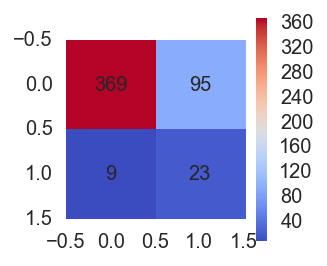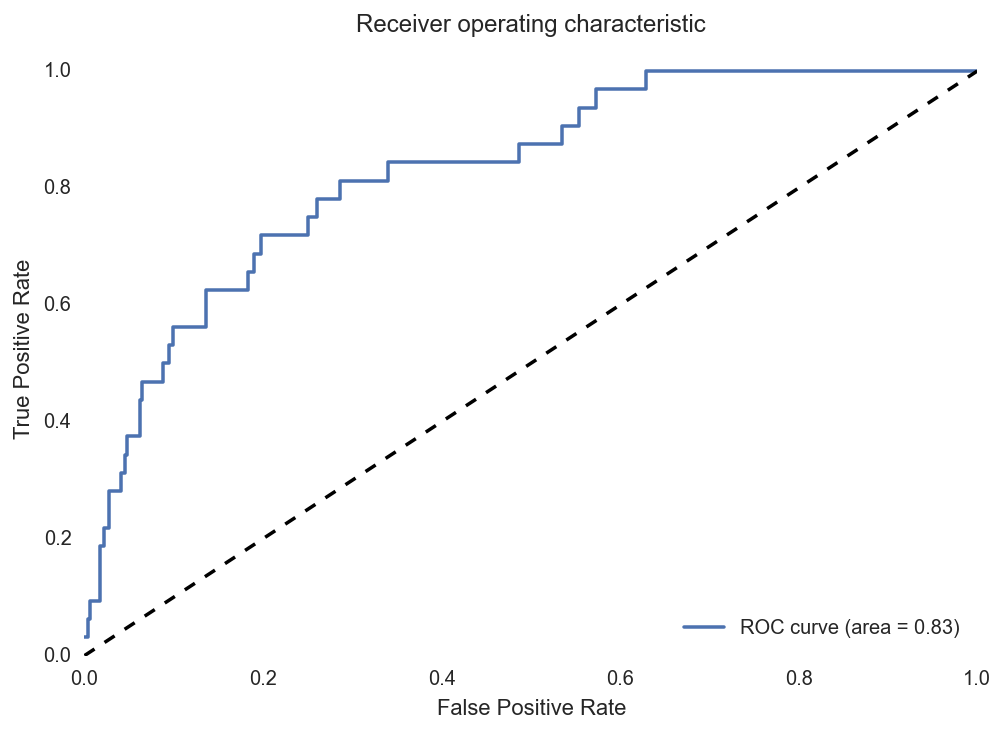### Final prediction

# Fit on learn
bestClf.fit(X_learn, y_learn)
y_final = bestClf.predict(X_final)

# Reorder prediction
bidders_y_final = pd.DataFrame(np.c_[X_ids[i_final], y_final], columns=['bidder_id', 'prediction'])
bidders_y_final[['prediction']] = bidders_y_final[['prediction']].astype(float)
bidders_list_final = pd.merge(bidders_list[['bidder_id']], bidders_y_final, how='left').fillna(0.0)

# Save to file
f = open('predictions_Lin_SVM_L1.csv', 'wb')
f.write(bidders_list_final.to_csv(index=False))
f.close()


## Random forest

Also a classifier well known to be great is the random forest. The problem is that it can have a lot of hyperparameters to tune. Basically all the parameters in the trees plus the ensemble settings such as number of estimators, but also random feature subspace size if you want, etc.

I chose to only CV grid search the number of estimators and the max depth of the tree. The idea of this code is again very close to the one of SVMs. But in this case I only used one grid search.

If I wanted a perfect there would definitely be things to factorize between those different classifiers (and scikit-learn is actually very good for this with their consistant interfaces across classifiers).

### Tuning the hyperparameters

# Coarse grid
HP_range = np.array([1, 2, 3, 4, 5, 6, 7, 8, 9, 10, 12, 15, 18, 20, 25, 30, 50, 100])
HP2_range = np.array([11, 21, 35, 51, 75, 101, 151, 201, 251, 301, 351, 401, 451, 501])
grid = GridSearchCV(sklearn.ensemble.RandomForestClassifier(n_estimators=300, max_depth=None,
max_features='auto', class_weight='auto'),
{'max_depth' : HP_range,
'n_estimators' : HP2_range},
cv=sklearn.cross_validation.StratifiedKFold(y_learn, 5), n_jobs=8, scoring='roc_auc')
grid.fit(X_learn, y_learn)

plotGridResults2D(HP_range, HP2_range, 'max depth', 'n estimators', grid.grid_scores_)
plt.show()

# Final res
bestClf = grid.best_estimator_
print bestClf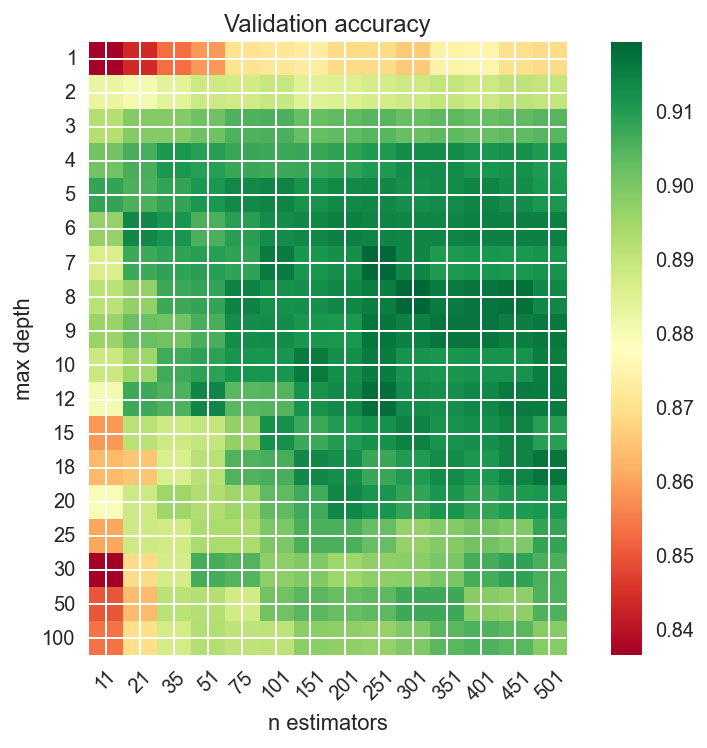RandomForestClassifier(bootstrap=True, class_weight='auto', criterion='gini',
max_depth=8, max_features='auto', max_leaf_nodes=None,
min_samples_leaf=1, min_samples_split=2,
min_weight_fraction_leaf=0.0, n_estimators=301, n_jobs=1,
oob_score=False, random_state=None, verbose=0,
warm_start=False)


### Testing the model

# Ok, I actally want to choose these paramters myself! I'm god here, I do what I want!
bestClf = sklearn.ensemble.RandomForestClassifier(max_depth=20, n_estimators=301,
max_features='auto', class_weight='auto')

# Learn on train for test
bestClf.fit(X_train, y_train)
y_pred = bestClf.predict(X_test)

# Classification report
print sklearn.metrics.classification_report(y_test, y_pred)
plotConfMap(sklearn.metrics.confusion_matrix(y_test, y_pred))
plt.show()

# Predict scores
y_score = bestClf.predict_proba(X_test)[:,1]

# ROC
fpr, tpr, _ = roc_curve(y_test, y_score)
roc_auc = auc(fpr, tpr)

plt.figure()
plt.plot(fpr, tpr, label='ROC curve (area = %0.2f)' % roc_auc)
plt.plot([0, 1], [0, 1], 'k--')
plt.xlim([0.0, 1.0])
plt.ylim([0.0, 1.05])
plt.xlabel('False Positive Rate')
plt.ylabel('True Positive Rate')
plt.legend(loc="lower right")
plt.show()

             precision    recall  f1-score   support

0.0       0.94      1.00      0.97       464
1.0       0.83      0.16      0.26        32

avg / total       0.94      0.94      0.93       496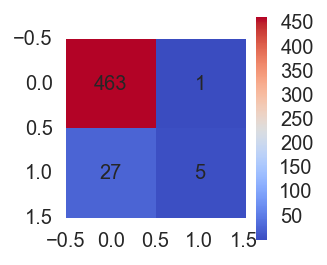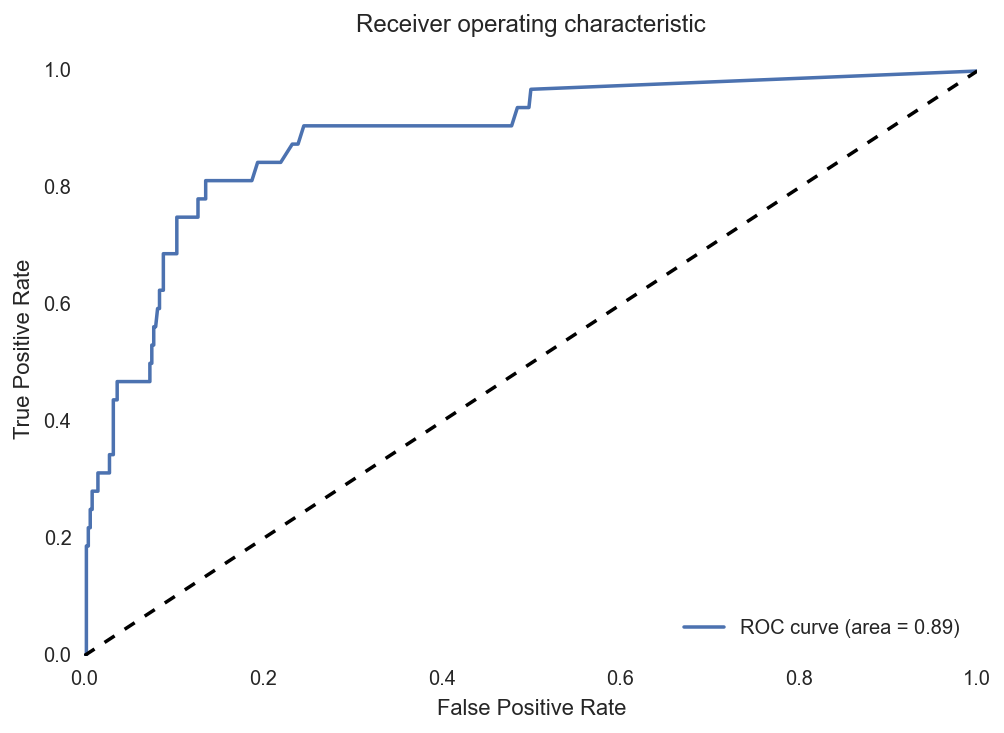bestClf.fit(X_learn, y_learn)
y_final = bestClf.predict_proba(X_final)[:,1]

bidders_y_final = pd.DataFrame(np.c_[X_ids[i_final], y_final], columns=['bidder_id', 'prediction'])
bidders_y_final[['prediction']] = bidders_y_final[['prediction']].astype(float)
bidders_list_final = pd.merge(bidders_list[['bidder_id']], bidders_y_final, how='left').fillna(0.0)

f = open('predictions_RF_2.0.csv', 'wb')
f.write(bidders_list_final.to_csv(index=False))
f.close()


Ok I also tried AdaBoost which is also a nice ensemble technique but it didn’t gave particularly better results than random forest. I think you got the idea of my code so I spare you the code for AdaBoost…

# Conclusion

I finally submitted predictions of my random forest for evaluation on the private leaderboard. I think this competition was more about feature engeneering than the pure Machine Learning part.

There are a lot of things I could have improved in my work. First, I could have used more complex features. Some good examples can be found for example on Kaggle’s forum of the competition “share your secret sauce” thread, or other similar threads. I think more complex time series analysis could have been done on bids times. I did not tried to clean the dataset, but I think that removing some wierd points from the training dataset might have been a good thing. Also I think there might be interesting ways to deal with the imbalance of the dataset (other than class weighting that I did of course), but I didn’t found time to dig into this. Finally regarding the pure Machine Learning part, using ensemble of different classifiers like some people did could also have improved the results.

Anyway, I am quite surprised of the good results I got with so simple features and model compared to what some other people did.

•